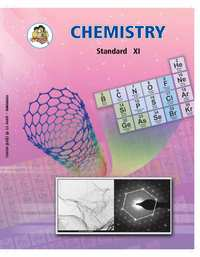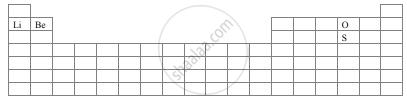# Balbharati solutions for Chemistry 11th Standard Maharashtra State Board chapter 7 - Modern Periodic Table [Latest edition]

#### Chapters## Chapter 7: Modern Periodic Table

Exercises
Exercises [Pages 3 - 109]

### Balbharati solutions for Chemistry 11th Standard Maharashtra State Board Chapter 7 Modern Periodic Table Exercises [Pages 3 - 109]

Exercises | Q 1. (A) | Page 108

Explain the following.

The elements Li, B, C, Be and N have the electronegativities 1.0, 2.0, 1.5, and 3.0, respectively on the Pauling scale.

Exercises | Q 1. (B) | Page 108

Explain the following.

The atomic radii of Cl, I, and Br are 99, 133, and 114 pm, respectively.

Exercises | Q 1. (C) | Page 108

Explain the following.

The ionic radii of FΘ and Naare 133 and 98 pm, respectively.

Exercises | Q 1. (D) | Page 108

Explain the following.

13Al is a metal, 14Si is a metalloid and 15P is a nonmetal.

Exercises | Q 1. (E) | Page 108

Explain the following.

Cu forms coloured salts while Zn forms colourless salts.

Exercises | Q 2. (A) | Page 108

Write the outer electronic configuration of the following using the orbital notation method. Justify.

Ge (belongs to period 4 and group 14)

Exercises | Q 2. (B) | Page 108

Write the outer electronic configuration of the following using the orbital notation method. Justify.

Po (belongs to period 6 and group 16)

Exercises | Q 2. (C) | Page 108

Write the outer electronic configuration of the following using the orbital notation method. Justify.

Cu (belongs to period 4 and group 11)

Exercises | Q 3. (A) | Page 108

La belongs to group 3 while Hg belongs to group 12 and both belong to period 6 of the periodic table. Write down the general outer electronic configuration of the ten elements from La to Hg together using the orbital notation method.

Exercises | Q 3. (B) | Page 3

Ionization enthalpy of Li is 520 kJ mol-1 while that of F is 1681 kJ mol-1. Explain.

Exercises | Q 3. (C) | Page 108

Explain the screening effect with a suitable example.

Exercises | Q 3. (D) | Page 108

Why the second ionization enthalpy is greater than the first ionization enthalpy?

Exercises | Q 3. (E) | Page 108

Why the elements belonging to the same group do have similar chemical properties?

Exercises | Q 3. (F) | Page 108

Explain electronegativity and electron gain enthalpy. Which of the two can be measured experimentally?

Exercises | Q 4. (A) | Page 108

Choose the correct option.

Consider the elements B, Al, Mg, and K predict the correct order of metallic character:

• B > Al > Mg > K

• Al > Mg > B > K

• Mg > Al > K > B

• K > Mg > Al > B

Exercises | Q 4. (B) | Page 108

Choose the correct option.

In the modern periodic table, the period number indicates the:

• atomic number

• atomic mass

• principal quantum number

• azimuthal quantum number

Exercises | Q 4. (C) | Page 109

Choose the correct option.

The lanthanides are placed in the periodic table at

• left-hand side

• right-hand side

• middle

• bottom

Exercises | Q 4. (D) | Page 109

Choose the correct option.

If the valence shell electronic configuration is ns2np5, the element will belong to

• alkali metals

• halogens

• alkaline earth metals

• actinides

Exercises | Q 4. (E) | Page 109

Choose the correct option.

In which group of elements of the modern periodic table are halogen placed?

• 17

• 6

• 4

• 2

Exercises | Q 4. (F) | Page 109

Choose the correct option.

Which of the atomic number represent the s-block elements?

• 7, 15

• 3, 12

• 6, 14

• 9, 17

Exercises | Q 4. (G) | Page 109

Choose the correct option.

Which of the following pairs is NOT isoelectronic?

• Naand Na

• Mg2⊕ and Ne

• Al3⊕ and B3⊕

• P and N

Exercises | Q 4. (H) | Page 109

Choose the correct option.

Which of the following pair of elements has similar properties?

• 13, 31

• 11, 20

• 12, 10

• 21, 33

Exercises | Q 5. (A)(a) | Page 109

The electronic configuration of some element is given below:

1s2

In which group and period of the periodic table the element is placed?

Exercises | Q 5. (A)(b) | Page 109

The electronic configuration of some element is given below:

1s2 2s2 2p6

In which group and period of the periodic table the element is placed?

Exercises | Q 5.(B)(a) | Page 109

For the following pair, indicate which of the two species is of large size:

Fe2+ or Fe3+

Exercises | Q 5.(B)(b) | Page 109

For the following pair, indicate which of the two species is of large size:

Mg2+ or Ca2+

Exercises | Q 5. (C)(a) | Page 109

Select the smaller ion form the following pair:

K+, Li+

Exercises | Q 5. (C)(b) | Page 109

Select the smaller ion form the following pair:

N3–, F

Exercises | Q 5. (D) | Page 109

With the help of a diagram answer the questions are given below:1. Which atom should have smaller ionization energy, oxygen, or sulphur?
2. The lithium forms +1 ions while beryllium forms +2 ions?
Exercises | Q 5. (E)(a) | Page 109

Exercises | Q 5. (E)(b) | Page 109

Define electronegativity

Exercises | Q 5. (F) | Page 109

Compare chemical properties of metals and non-metals.

Exercises | Q 5. (G) | Page 109

What are the valence electrons?

Exercises | Q 5. (G) | Page 109

For s-block and p-block elements show that the number of valence electrons is equal to its group number.

Exercises | Q 5. (H) | Page 109

Define ionization energy.

Exercises | Q 5. (H) | Page 109

Name the factors on which ionization energy depends?

Exercises | Q 5. (H) | Page 109

How does ionization energy vary down the group and across a period?

Exercises | Q 5. (I) | Page 109

How the atomic size vary in a group and across a period? Explain with suitable example.

Exercises | Q 5. (J)(a) | Page 109

Give reason.

Alkali metals have low ionization energies.

Exercises | Q 5. (J)(b) | Page 109

Give reason.

Inert gases have exceptionally high ionization potential.

Exercises | Q 5. (J)(c) | Page 109

Give reason.

Fluorine has less electron affinity than chlorine.

Exercises | Q 5. (J)(d) | Page 109

Give reason.

Noble gases possess relatively large atomic size.

Exercises | Q 5. (K)(a) | Page 109

Consider the oxides Li2O, CO2, B2O3.

Which oxide would you expect to be the most basic?

Exercises | Q 5. (K)(b) | Page 109

Consider the oxides Li2O, CO2, B2O3.

Which oxide would be the most acidic?

Exercises | Q 5. (K)(c) | Page 109

Consider the oxides Li2O, CO2, B2O3.

Give the formula of an amphoteric oxide.

## Chapter 7: Modern Periodic Table

Exercises## Balbharati solutions for Chemistry 11th Standard Maharashtra State Board chapter 7 - Modern Periodic Table

Balbharati solutions for Chemistry 11th Standard Maharashtra State Board chapter 7 (Modern Periodic Table) include all questions with solution and detail explanation. This will clear students doubts about any question and improve application skills while preparing for board exams. The detailed, step-by-step solutions will help you understand the concepts better and clear your confusions, if any. Shaalaa.com has the Maharashtra State Board Chemistry 11th Standard Maharashtra State Board solutions in a manner that help students grasp basic concepts better and faster.

Further, we at Shaalaa.com provide such solutions so that students can prepare for written exams. Balbharati textbook solutions can be a core help for self-study and acts as a perfect self-help guidance for students.

Concepts covered in Chemistry 11th Standard Maharashtra State Board chapter 7 Modern Periodic Table are Introduction of Periodic Table, Structure of the Modern Periodic Table, Periodic Table and Electronic Configuration, Blockwise Characteristics of Elements, Periodic Trends in Elemental Properties.

Using Balbharati 11th solutions Modern Periodic Table exercise by students are an easy way to prepare for the exams, as they involve solutions arranged chapter-wise also page wise. The questions involved in Balbharati Solutions are important questions that can be asked in the final exam. Maximum students of Maharashtra State Board 11th prefer Balbharati Textbook Solutions to score more in exam.

Get the free view of chapter 7 Modern Periodic Table 11th extra questions for Chemistry 11th Standard Maharashtra State Board and can use Shaalaa.com to keep it handy for your exam preparation